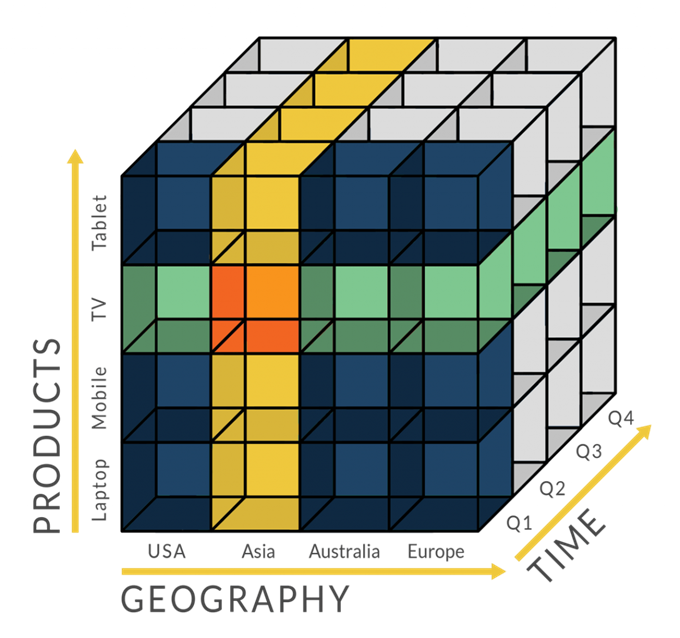# 生存分析从概念到实战

## 什么是生存分析？

### 解决方案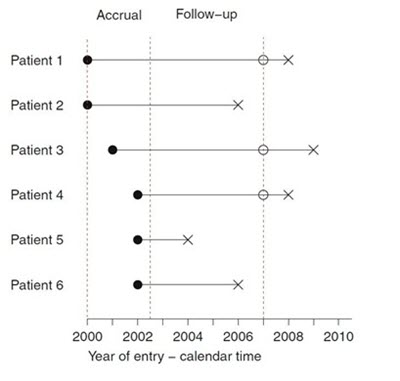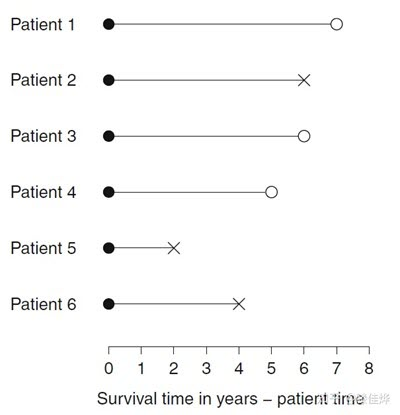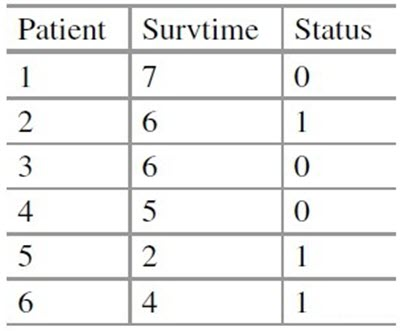• $t_i$代表了跟进时间（following-up time），是生存时间T_i与删失时间C_i的较小值
• $\delta _i$代表了状态（status），$if t_i=T_i, \delta _i =1; ift_i=C_,i \delta _i =0.$
• $x_i$代表了协变量（covariates），包括感兴趣的其他变量（如身高血压肺活量……）

### 基本概念

• 起始事件(initial event)：反应生存时间起始特征的事件，如疾病确诊、某种疾病治疗开始等。
• 失效事件(failure event)：常被简称为事件，研究者规定的终点结局，医学研究中可以是患者死亡，也可以是疾病的发生、某种治疗的反应、疾病的复发等。在生存分析随访研究过程中，一部分研究对象可观察到死亡，可以得到准确的生存时间，它提供的信息是完全的，这种事件称为失效事件，也称之为死亡事件、终点事件。

• 服药→痊愈
• 手术切除→死亡
• 染毒→死亡
• 化疗→缓解
• 缓解→复发

• 分布类型不易确定。一般不服从正态分布，多数情况下不服从任何规则的分布类型。
• 影响因素多而复杂且不易控制。
• 根据研究对象的结局，生存时间数据可分为两种类型：
• 完全数据(Completed Data)：从观察起点到发生死亡事件所经历的时间。
• 不完全数据(Incomplete Data)：生存时间观察过程的截止不是由于死亡事件，而是由其他原因引起的。
• 不完全数据分为：删失数据(censored Data)和截尾数据(truncated Data)。
• 不完全主要原因：
• 失访：指失去联系；
• 退出：死于非研究因素或非处理因素而退出研究；
• 终止：设计时规定的时间已到而终止观察，但研究对象仍然存活。

• 删失分类：
• 左删失（left censored）：研究对象在某一时刻开始接受观察，但是在该时间点之前，研究所感兴趣的事件已经发生，无法明确具体时间。
• 右删失（right censored）：在进行随访观察中，研究对象观察的起始时间已知，但终点事件发生的时间未知，无法获取具体的生存时间，只知道生存时间大于观察时间。
• 区间删失（interval censored）：在实际的研究中，如果不能够进行连续的观察随访，只能预先设定观察时间点，研究人员仅能知道每个研究对象在两次随访区间内是否发生终点事件，而不知道准确的发生时间。
• 截尾是所有样本的综合特性，指的是观察的总体是有偏的，只有当事件的失效时间出现在观测区间内，我们才能知道这个事件及其观测数据的存在。
• 左截尾（left truncation）：只能观测到一个时间点之后发生的失效事件。左截尾时间点之前发生的失效事件不知情/不关心（如样本来自退休中心，都是>60岁的老人）。
• 右截尾（right truncation）：只能观测到一个时间点之前发生的失效事件。右截尾时间点之后发生的失效事件不知情/不关心

• 若含有删失数据，须分时段计算生存概率。假定观察对象在各个时段的生存时间独立，应用概率乘法定理将分时段的概率相乘得到生存率。
• 生存率与条件生存概率不同。条件生存概率是单个时段的结果，而生存率实质上是累积条件生存概率（cumulative probability of survival ），是多个时段的累积结果。例如，3 年生存率是第1 年存活，第2 年也存活，第3 年还存活的可能性。
• 生存率s(t)的估计方法有参数法和非参数法。常用非参数法，非参数法主要有二个，即，乘积极限法与寿命表法，乘积极限法主要用于观察例数较少而未分组的生存资料，寿命表法适用于观察例数较多而分组的资料，不同的分组寿命表法的计算结果亦会不同，当分组资料中每一个分组区间中最多只有1个观察值时，寿命表法的计算结果与乘积极限法完全相同。
• 生存曲线(survival curve)：以观察（随访）时间为横轴，以生存率为纵轴，将各个时间点所对应的生存率连接在一起的曲线图。生存曲线是一条下降的曲线，分析时应注意曲线的高度和下降的坡度。平缓的生存曲线表示高生
• 中位生存时间（Median Survival Time）/平均生存时间（Mean Survival Time）：中位生存时间又称半数生存期，表示恰好一半个体未发生失效事件的时间，生存曲线上纵轴50%对应的时间。平均生存时间则表示生存曲线下的面积。

### 应用场景

• 研究某病治疗后的复发情况，复发就是“死亡”，未复发就是“生存”。只要有复发的结局（是否复发）以及从治疗后到复发的时间，就可以用生存分析。
• 研究工作后升迁的因素有哪些，升迁就是“死亡”，未升迁就是“生存”。只要有升迁的结局（是否升迁）以及从开始工作到升迁的时间，就可以用生存分析。
• 研究戒烟后复吸的因素，复吸就是“死亡”，未复吸就是“生存”。只要有复吸的结局（是否复吸）以及从戒烟工作到复吸的时间，就可以用生存分析。

## 生存分析常用方法

• 组间比较：t检验、方差分析
• 多因素分析：线性回归

• 组间比较：Kaplan-Meier
• 多因素分析：Cox回归

• 生存概率，即 Survival probability，指的是研究对象从试验开始直到某个特定时间点仍然存活的概率，可见它是一个对时间t的函数，我们定义之为 S(t)；
• 风险概率，即 Hazard probability ，指的是研究对象从试验开始到某个特定时间 t 之前存活，但在 t 时间点发生观测事件如死亡的概率，它也是对时间 t 的函数，定义为 H(t)。

### Kaplan-Meier模型

Kaplan Meier，是一种单因素生存分析。它可用于研究1个因素对于生存时间的影响，在医疗领域中使用广泛。

Kaplan–Meier 方法，该方法是由 Kaplan 和 Meier 与 1958 年共同提出的，为理解方法的细节，我们先看下一张表(原文链接)。本例中我们以死亡作为观测事件，这张表也叫做 life table.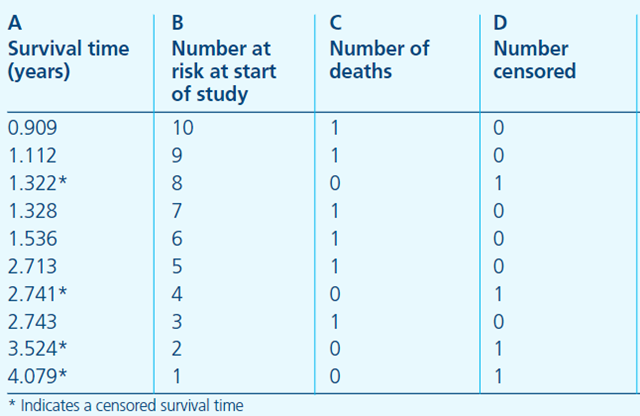• A 列是从试验开始起，持续的观测时间，星号代表在该时间有删失数据发生；
• B 列是指在 A 列对应的时间开始之前所有存活的研究对象个数，也可以叫做 at risk 的人数，表示当前具有死亡风险的有效人群，是排除了已经死亡和删失的数据之后剩余的人数；
• C 列为恰好在 A 列对应的时间死亡的人数，
• D 列即表明在该时间点删失的个数。第一行则可以解读为，在909 年这个时间点之前，本来有 10 个患者，在 0.909 这个时间点（或其之后的一小段时间区间）死亡了一个人，没有删失数据，意味着还剩 9 人；随后，只要有新增死亡或删失数据，则在表中新建一行，记录时间和人数。

P(存活至1.536) = P(0.909时不死亡) * P(1.112时不死亡) * P(1.322时不死亡) * P(1.328时不死亡) * P(1.536时不死亡)

P(0.909时不死亡) = 1 – P(0.909时死亡) = 1 – (0.909时死亡的人数)/(0.909之前的所有人数) = 1 – 1/10 = 0.9
P(1.112时不死亡) = 1 – P(1.112时死亡) = 1 – (1.112时死亡的人数)/(1.112之前的所有人数) = 1 – 1/9 = 0.89

P(1.322时不死亡) = 1 – P(1.322时死亡) = 1 – (1.322时死亡的人数)/(1.322之前的所有人数) = 1 – 0/9 = 1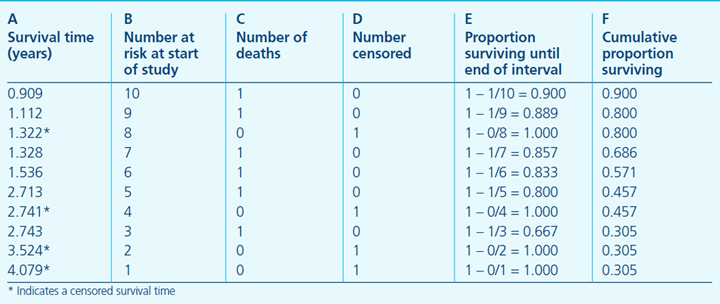$$S(t_i)=S(t_{i-1}(1-\frac{d_i}{n_i})$$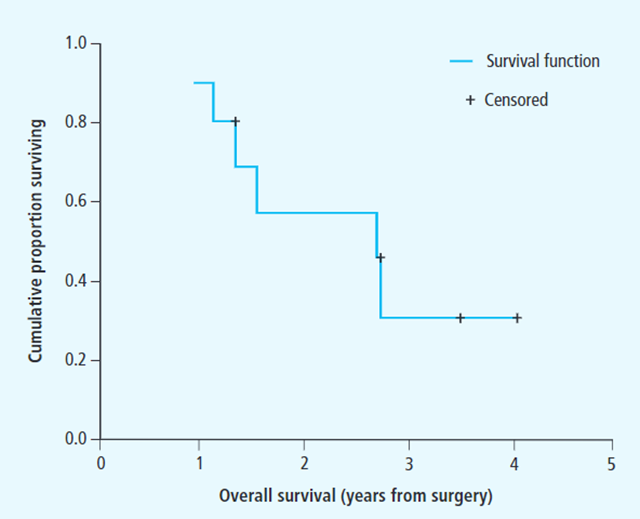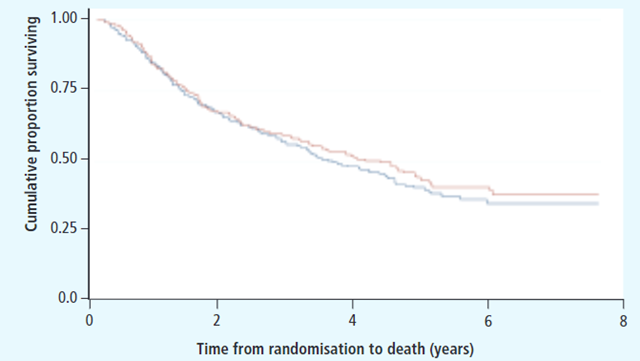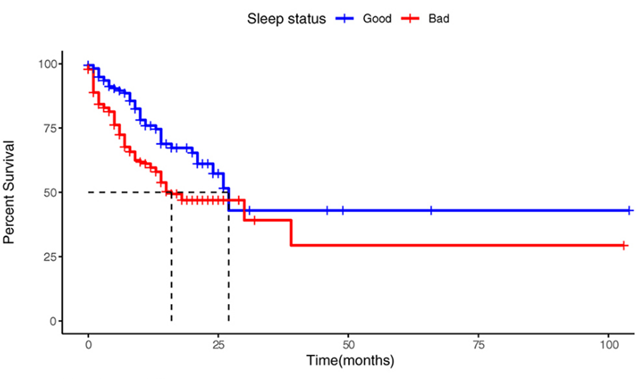Logrank 方法是由 Nathan Mantel 最初提出的，它是一种非参数检验，中文翻译为对数秩检验，主要用来比较两组样本的生存时间分布的差异。Logrank 实际计算形式可能有不同的变体，我们这里介绍一种版本，实际上是卡方检验的一种应用场景。Logrank 检验的零假设是指两组的生存时间分布完全一致，当我们通过计算拒绝零假设时，就可以认为两组的生存时间分布存在统计学差异。我们可以通过以下公式计算某组病人在某个时间点的期望死亡人数：

$$E_{1t} = N_{1t}*(O_t/N_t)$$

• $E_{1t}$是指第一组中，在时刻 t，期望死亡人数；
• $N_{1t}$指第一组中 t 时刻 at risk的人数，即 t 之前的存活人数；
• $O_t$则指两组（第一组和第二组）总的观测到的实际死亡人数；
• $N_t$指两组总的 at risk 的人数，或 t 时间之前两组的总人数。

$$\chi^2=\sum \frac{(\sum O_{j t}-\sum E_{jt})^2}{\sum E_{jt}}$$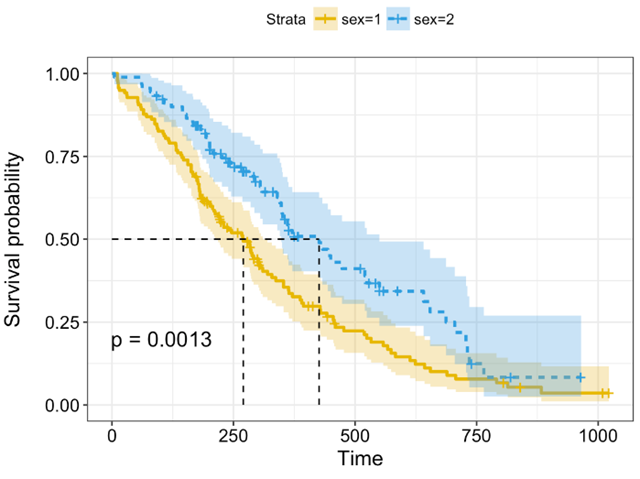\begin{aligned}&\widehat{S}(t) \pm z_{\alpha / 2} \sqrt{\widehat{\operatorname{Var}}[\widehat{S}(t)]} \quad \text { where } \\ &\widehat{\operatorname{Var}}[\widehat{S}(t)]=\widehat{S}(t)^2 \sum_{t_i \leq t} \frac{d_i}{n_i(n_i-d_i)} \end{aligned}

• LogRank检验各时点的权重均为“1”。就是不考虑各观察时点开始时存活的人数对统计模型的影响。也就是每个时点死亡情况的变化对整个模型的贡献是一样的。
• Breslow检验则在Log Rank检验的基础上增加了权重，并设置权重为各时点开始时存活的人数。也就是开始存活人数多的时点死亡情况的变化对整个模型的贡献较大，而开始存活人数少的时点死亡情况的变化对整个模型的贡献较小。
• Tarone-Ware检验是权重的取值方法介于以上两种方法之间，设置权重为各时点开始时存活的人数的平方根。同样是开始存活人数多的时点死亡情况的变化对整个模型的贡献较大，而开始存活人数少的时点死亡情况的变化对整个模型的贡献较小。只是开始存活人数多的时点对整个模型的贡献不如Breslow检验大。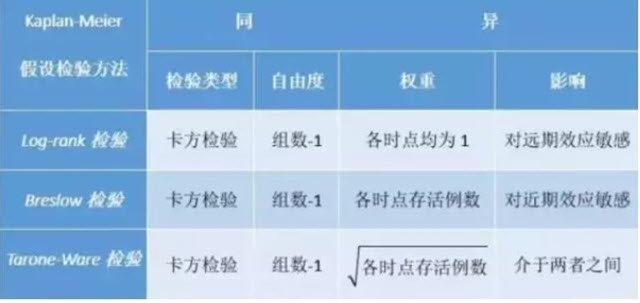### Cox回归

Cox 模型是一种半参数模型，因为它的公式中既包括参数模型又包括非参数模型。简单说下参数模型和非参数模型的相同与区别。相同点是它们都是用来描述某种数据分布情况的；不同点在于，参数模型的参数是有限维度的，即有限个参数就可以表示模型分布，比如正态分布里的均值和标准差；而非参数模型的参数则属于某个无限维的空间，无法用有限参数来表示，不同的数据会得到不同的分布估计，比如决策树、随机森林等等，我们无法用有限的参数来表示所有可能的分布情况。来看看 Cox 模型的公式就知道为什么 Cox 模型是一种半参数模型了。

$$h(t)=h_0(t)\times \exp(b_1x_1+b_2x_2+…+b_px_p)$$

$$\text{hazard ratio}=\frac{h_0(t)*\exp(b_1(x_1+1)+b_2x_2+…+b_px_p)}{b_0(t)*\exp(b_1x_1+b_2x_2+…+b_px_p)} = \frac{\exp(b_1(x_1+1)+b_2x_2+…+b_px_p}{\exp(b_1x_1+b_2x_2+…+b_px_p)}=\exp(b_1)$$

• 如果$b_1>0$，则 $\exp(b_1)>1$，意味着年龄 +1，死亡风险增加；
• 如果$b_1<0$, 则 $\exp(b_1)<1$，意味着年龄 +1，死亡风险降低；
• 如果$b_1=0$，则 $\exp(b_1)=1$，意味着年龄变化对死亡风险不起作用。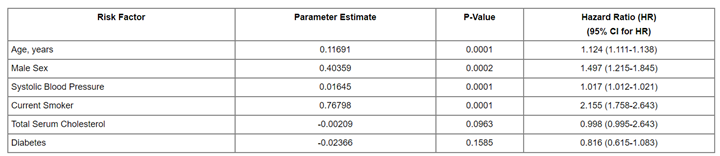\begin{aligned} h(t) &=h_0(t) \exp (\beta X) \\ H(t) &=H 0(t) \exp (\beta X) \\ \log H(t) &=\log H_0(t)+\beta X \\ \log [-\log S(t)] &=\log [-\log S_0(t)] + \beta X \end{aligned}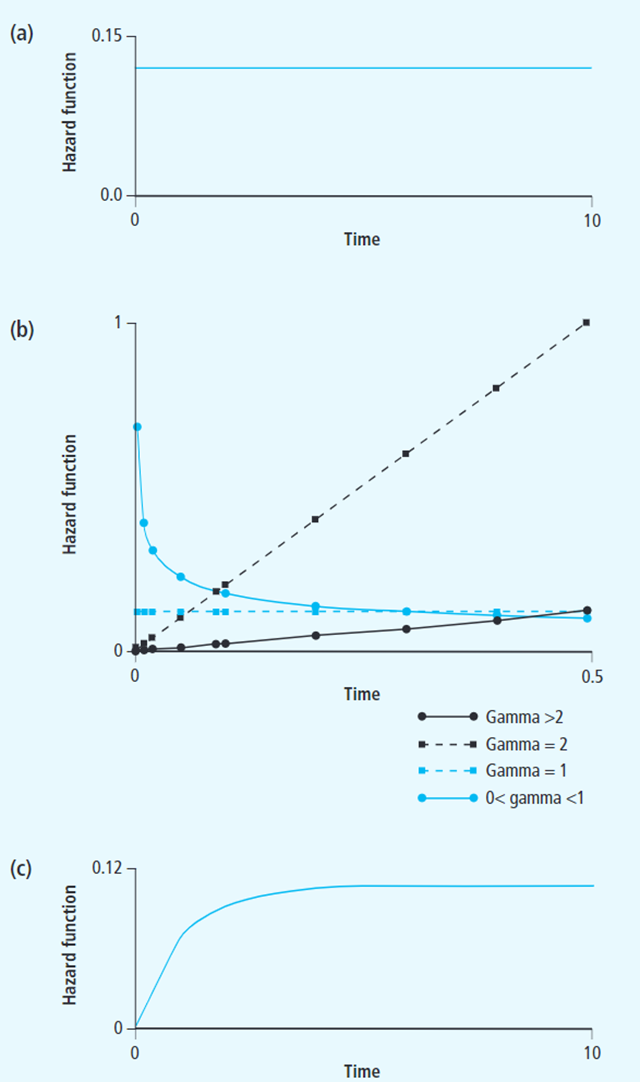## 使用PySurvival分析用户流失

• Python版本：官方说是7~3.7，实际上的3.8也可以。但是超过3.8就会存在问题
• Windows上安装一直不成功。所以在WLS中进行的安装

PySurvival 内部自带了一个数据集，我们就是用内部数据来分析。自带的数据来自一家Saas服务公司的客户数据，该公司的主要商业模式是每月收费。

1、加载需要使用到的包（依赖）

import pandas as pd
import numpy as np
import matplotlib.pyplot as plt
from sklearn.model_selection import train_test_split
from pysurvival.datasets import Dataset
from pysurvival.utils.display import correlation_matrix, compare_to_actual, integrated_brier_score, create_risk_groups
from pysurvival.utils.metrics import concordance_index
from pysurvival.models.survival_forest import ConditionalSurvivalForestModel

import warnings
warnings.filterwarnings("ignore")

pd.set_option("display.precision",2)
np.set_printoptions(precision=2, suppress=True)
pd.options.display.float_format = '{:,.0f}'.format


2、加载并查看数据

df0 = Dataset("churn").load()
df0.tail()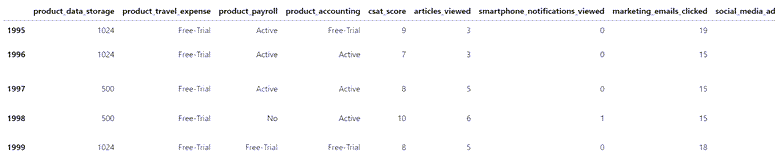<class 'pandas.core.frame.DataFrame'>
RangeIndex: 2000 entries, 0 to 1999
Data columns (total 14 columns):
#   Column                           Non-Null Count  Dtype
---  ------                           --------------  -----
0   product_data_storage             2000 non-null   int64
1   product_travel_expense           2000 non-null   object
2   product_payroll                  2000 non-null   object
3   product_accounting               2000 non-null   object
4   csat_score                       2000 non-null   int64
5   articles_viewed                  2000 non-null   int64
7   marketing_emails_clicked         2000 non-null   int64
9   minutes_customer_support         2000 non-null   float64
10  company_size                     2000 non-null   object
11  us_region                        2000 non-null   object
12  months_active                    2000 non-null   float64
13  churned                          2000 non-null   float64
dtypes: float64(3), int64(6), object(5)
memory usage: 218.9+ KB


 特征类别 特征名称 类型 描述 Time months_active numerical Number of months since the customer started his/her subscription Event churned categorical Specifies if the customer stopped doing business with the company Products product_data_storage numerical Amount of cloud data storage purchased in Gigabytes Products product_travel_expense categorical Indicates if the customer is actively using and paying for the Travel and Expense management services or not. (‘Active’, ‘Free-Trial’, ‘No’) Products product_payroll categorical Indicates if the customer is actively using and paying for the Payroll management services or not. (‘Active’, ‘Free-Trial’, ‘No’) Products product_accounting categorical Indicates if the customer is actively using and paying for the Accounting services or not. (‘Active’, ‘Free-Trial’, ‘No’) Satisfaction csat_score numerical Customer Satisfaction Score (CSAT) is the measure of how products and services supplied by the company meet customer expectations. Satisfaction minutes_customer_support numerical Minutes the customer spent on the phone with the company customer support Marketing articles_viewed numerical Number of articles the customer viewed on the company website. Marketing smartphone_notifications_viewed numerical Number of smartphone notifications the customer viewed Marketing marketing_emails_clicked numerical Number of marketing emails the customer opened on Marketing social_media_ads_viewed numerical Number of social media ads the customer viewed Customer information company_size categorical Size of the company Customer information us_region categorical Region of the US where the customer’s headquarter is located

• “churned”，用于识别过去未续订的客户
• “months_active”，它添加了一个时间维度，我们希望沿着该维度跟踪客户流失风险

df0.describe()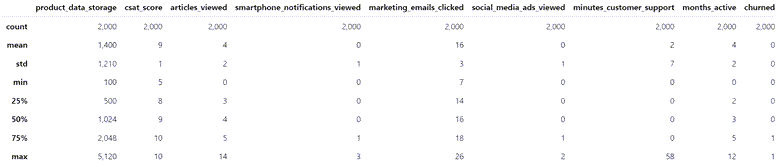def plot_nums(df):
numcols = list(df.dtypes[df.dtypes != np.object].index)
for col in numcols:
fig, ((ax1, ax2)) = plt.subplots(1, 2,  figsize=(15, 4))
x = df[numcols].values
ax1.boxplot(x)
ax1.set_title("{}".format(col))
ax2.hist(x, bins=20)
ax2.set_title("{}".format(col))
plt.show()

plot_nums(df0)


df0.describe(include='object').T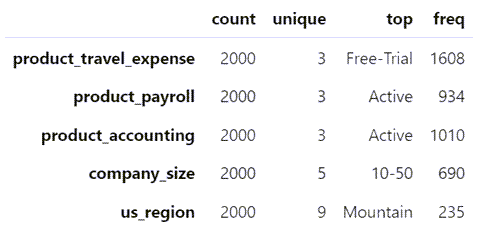# backup the original data before modifications
df1 = df0.copy()

# create numerical columns from categories via one-hot encoding
catcols = list(df1.dtypes[df1.dtypes == np.object].index)
df1 = pd.get_dummies(df1, columns=catcols, drop_first=True)
df1.info()

<class 'pandas.core.frame.DataFrame'>
RangeIndex: 2000 entries, 0 to 1999
Data columns (total 27 columns):
#   Column                             Non-Null Count  Dtype
---  ------                             --------------  -----
0   product_data_storage               2000 non-null   int64
1   csat_score                         2000 non-null   int64
2   articles_viewed                    2000 non-null   int64
4   marketing_emails_clicked           2000 non-null   int64
6   minutes_customer_support           2000 non-null   float64
7   months_active                      2000 non-null   float64
8   churned                            2000 non-null   float64
9   product_travel_expense_Free-Trial  2000 non-null   uint8
10  product_travel_expense_No          2000 non-null   uint8
11  product_payroll_Free-Trial         2000 non-null   uint8
12  product_payroll_No                 2000 non-null   uint8
13  product_accounting_Free-Trial      2000 non-null   uint8
14  product_accounting_No              2000 non-null   uint8
15  company_size_10-50                 2000 non-null   uint8
16  company_size_100-250               2000 non-null   uint8
17  company_size_50-100                2000 non-null   uint8
18  company_size_self-employed         2000 non-null   uint8
19  us_region_East South Central       2000 non-null   uint8
20  us_region_Middle Atlantic          2000 non-null   uint8
21  us_region_Mountain                 2000 non-null   uint8
22  us_region_New England              2000 non-null   uint8
23  us_region_Pacific                  2000 non-null   uint8
24  us_region_South Atlantic           2000 non-null   uint8
25  us_region_West North Central       2000 non-null   uint8
26  us_region_West South Central       2000 non-null   uint8
dtypes: float64(3), int64(6), uint8(18)
memory usage: 175.9 KB


df1.select_dtypes(exclude="object").nunique()

product_data_storage                   6
csat_score                             6
articles_viewed                       13
marketing_emails_clicked              20
minutes_customer_support             217
months_active                         13
churned                                2
product_travel_expense_Free-Trial      2
product_travel_expense_No              2
product_payroll_Free-Trial             2
product_payroll_No                     2
product_accounting_Free-Trial          2
product_accounting_No                  2
company_size_10-50                     2
company_size_100-250                   2
company_size_50-100                    2
company_size_self-employed             2
us_region_East South Central           2
us_region_Middle Atlantic              2
us_region_Mountain                     2
us_region_New England                  2
us_region_Pacific                      2
us_region_South Atlantic               2
us_region_West North Central           2
us_region_West South Central           2
dtype: int64


3、数据加工处理：确定目标列和在特征中取出目标

• “事件”——在我们的示例中，“churned”= 1 或 0
• 时间维度：“months_active”

# target variables: months_active and churns
time_column = 'months_active'
event_column = 'churned'

# list of feature columns: excluding the target columns
features = np.setdiff1d(df1.columns, [time_column, event_column]).tolist()
X = df1[features]
X.tail()


4、查看特征间的相关性

# correlation matrix of the features
correlation_matrix(df1[features], figure_size=(30,15), text_fontsize=10)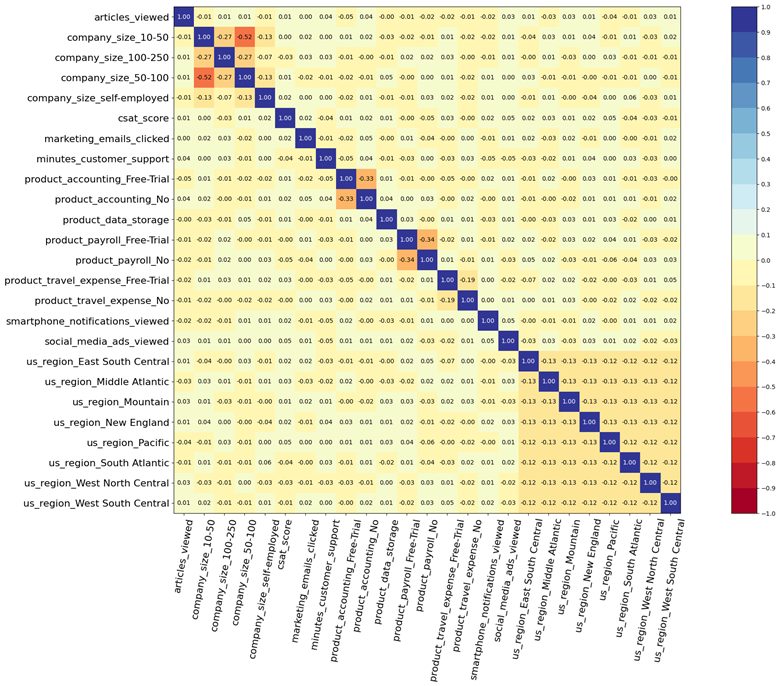5、准备模型数据

# sklearn: train vs test split
N = df1.shape
idx_train, idx_test = train_test_split(range(N), test_size = 0.35)
df_train = df1.loc[idx_train].reset_index(drop = True)
df_test  = df1.loc[idx_test].reset_index(drop = True)

# features, times (months active), and churn events: isolate the X, T and E variables
X_train, X_test = df_train[features], df_test[features]
T_train, T_test = df_train[time_column], df_test[time_column]       # when did the Churn event occur?
E_train, E_test = df_train[event_column], df_test[event_column]     # vdid the Churn event occur?


6、训练模型

• 传统的回归模型对两个数据数组 X 和 y 进行操作：回归量 X 和目标向量 y。
• 而生存模型对三个变量 X、E 和 T 进行操作：
• 特征数组 X（由代表属性或客户资料的列组成）
• 二进制事件指示符向量 E（1 或 0），表示是否已为客户记录流失事件；
• 时间向量 T = min(t, c)，其中 t 表示事件时间，c 表示审查时间

# fitting a survival forest model
model = ConditionalSurvivalForestModel(num_trees=200)
model.fit(
X_train,
T_train,
E_train,
max_features="sqrt",    # number of features randomly chosen at each split (int, float, "sqrt", "log2", or "all")
max_depth=5,            #
min_node_size=20,       # minimum number of samples at leaf node
alpha=0.05,             # significance threshold to allow splitting
seed=42,                # seed for random variable generator
sample_size_pct=0.63,   # % of original samples used in each tree building
num_threads=-1          # number of jobs to run; if -1, then all available cores will be used
)


4、测试模型质量

C-index，英文名全称concordance index，中文里有人翻译成一致性指数，最早是由范德堡大学（Vanderbilt University）生物统计教教授Frank E Harrell Jr 1996年提出，主要用于计算生存分析中的COX模型预测值与真实之间的区分度（discrimination），和大家熟悉的AUC其实是差不多的；在评价肿瘤患者预后模型的预测精度中用的比较多。

C-index的计算方法是把所研究的资料中的所有研究对象随机地两两组成对子，以生存分析为例,两个病人如果生存时间较长的一位其预测生存时间长于另一位,或预测的生存概率高的一位的生存时间长于另一位,则称之为预测结果与实际结果相符，称之为一致。

C-index在0.5-1之间（任意配对随机情况下一致与不一致刚好是0.5的概率）。0.5为完全不一致,说明该模型没有预测作用,1为完全一致,说明该模型预测结果与实际完全一致。一般情况下C-index：

• 在50-0.70为准确度较低
• 在71-0.90之间为准确度中等
• 高于90则为高准确度

Brier Score (mean squared error)跟L2 loss 很像。

$$BS=\frac{1}{N} \sum_{t=1}^N (\hat{y}_t-y_t)^2$$

$$L2loss= \sum_{t=1}^N (\hat{y}_t-y_t)^2$$

Brier Score 的出来的范围 是 [0,1] 之间，然后Brier Score 越小则模型准确率越高。

$$IBS (\tau, V_U, \hat{S}(\cdot \mid \cdot))=\frac{1}{\tau} \int_0^\tau B S_t (V_U, \hat{S}(t \mid \cdot))dt$$

# testing: model quality - prediction error measures by concordance index and Brier score
ci = concordance_index(model, X_test, T_test, E_test)
print("concordance index: {:.2f}".format(ci))

ibs = integrated_brier_score(model, X_test, T_test, E_test, t_max=12, figure_size=(15,5))
print("integrated Brier score: {:.2f}".format(ibs))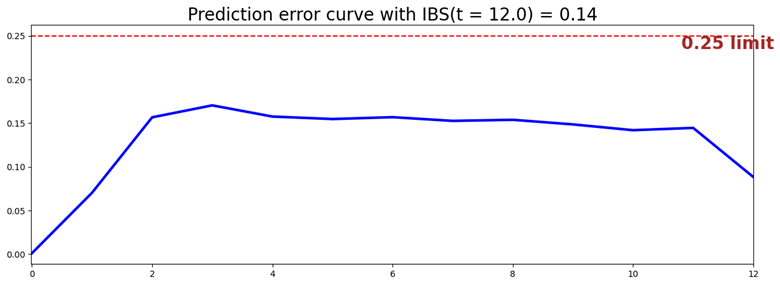• concordance index: 0.83
• integrated Brier score: 0.14

PySurvival 的compare_to_actual 方法沿时间轴绘制风险客户的预测和实际数量。它还计算三个准确度指标，RMSE、MAE 和中值绝对误差。在内部，它计算 Kaplan-Meier 估计量以确定源数据的实际生存函数。

# testing: model quality - actual vs predicted churn events
res = compare_to_actual(
model,
X_test,
T_test,
E_test,
is_at_risk = False,       # True: return the expected number of customers at risk
figure_size=(16, 6),
metrics = ["rmse", "mean", "median"])   # root mean square error, mean abs error, median abs error
print("accuracy metrics:")
_ = [print(k,":",f'{v:.2f}') for k,v in res.items()]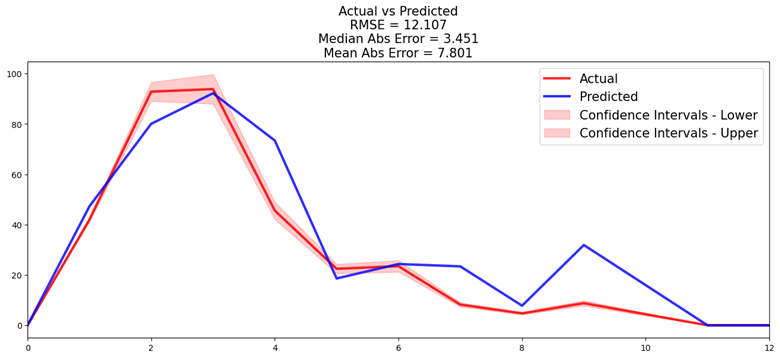accuracy metrics:
root_mean_squared_error : 12.11
median_absolute_error : 3.45
mean_absolute_error : 7.80

6、模型结果：特征重要性

“pct_importance”列标识在 0 到 1 的范围内校准相对重要性。相对重要性加起来为 1.0。

• csat_score——通过营销调查衡量的客户满意度得分——与客户流失风险密切相关。
• product_payroll_No和product_accounting_No，不清楚这整个维度具体什么意思。
• minutes_customer_support表示那些在取消订阅之前经常向支持热线投诉的客户存在高流失风险。
# model results: variable importance
# positive: increase the risk; negative: alleviate the risk
pd.options.display.float_format = '{:,.3f}'.format
model.variable_importance_table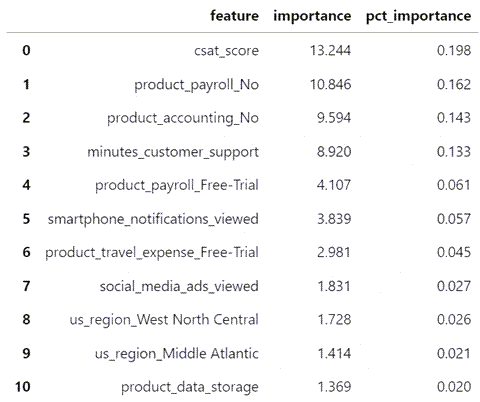7、预测个体的生存函数、危险函数和风险评分

# survival function, hazard function and risk score for a randomly drawn individual
k = np.random.choice(df1.index)

# t = number of time periods for which to show the probabilities
# if t = None, then t = maximum number of "months active" in dataset

svf = model.predict_survival(X.values[k,:], t=None)     # survival function over time
hzf = model.predict_hazard(X.values[k,:], t=None)       # hazard function over time
risk = model.predict_risk(X.values[k,:])        # risk score (scalar)

df_risk = pd.DataFrame()
df_risk["svf"] = svf.flatten().tolist()
df_risk["hzf"] = hzf.flatten().tolist()
df_risk.insert(2, "risk", risk.item())
print("risk score, and survival and hazard functions over time, for customer", k, ":")
df_risk


8、计算每个用户的在4个时期的生存函数和风险分值

# compute every customer's survival function and risk score for 4 chosen periods (active months)
T = [1,3,6,12]
for t in T:
svf = model.predict_survival(X.values, t=t)     # survival function over time
df1["svf" + str(t)] = svf
df1["risk"] = model.predict_risk(X.values)            # risk score
df1.tail()

# predictions: customer quartiles, grouped by their risk scores
q1 = df1["risk"].quantile(0.25)
q2 = df1["risk"].quantile(0.50)
q3 = df1["risk"].quantile(0.75)
q4 = df1["risk"].max()
risk_groups = create_risk_groups(
model=model,
X=X_test,
use_log = False,
num_bins=30,
figure_size=(20, 4),
q1={'lower_bound':0,  'upper_bound':q1, 'color':'red'},
q2={'lower_bound':q1, 'upper_bound':q2, 'color':'green'},
q3={'lower_bound':q2, 'upper_bound':q3, 'color':'blue'},
q4={'lower_bound':q3, 'upper_bound':q4, 'color':'black'}
)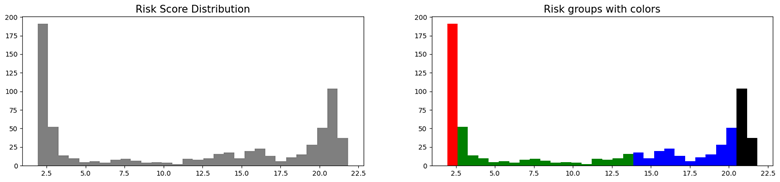## 使用Lifelines进行生存分析实战

### lifelines简介

lifelines是Python中使用较多的生存分析包，其核心功能主要有：

 模块 描述 类型 方法 survival function 研究对象从试验开始直到某个特定时间点仍然存活的概率 参数估计 Exponential, Log-Logistic, Log-Normal and Splines 非参数估计 Kaplan-Meier估计 cumulative hazard 风险函数的估计值 参数估计 Exponential, Log-Logistic, Log-Normal and Splines 非参数估计 Nelson-Aalen估计 Survival regression 会加入额外的协变量(如年龄、国家等)与另一个变量进行回归 比例回归 Cox 比例风险回归模型，指数回归模型 ，Weibull回归模型，Poisson回归模型 非比例回归 含参数与半参模型：Aalen’s Additive model 模型 、 CoxTimeVarying时变模型、AFT(accelerated failure time model)加速失效模型

### 数据预览

• “Tenure”：观察数据时他们成为客户的时间
• “Churn”：观察数据时客户是否离开

1、加载数据看一些基础的数据情况

import pandas as pd
data.info()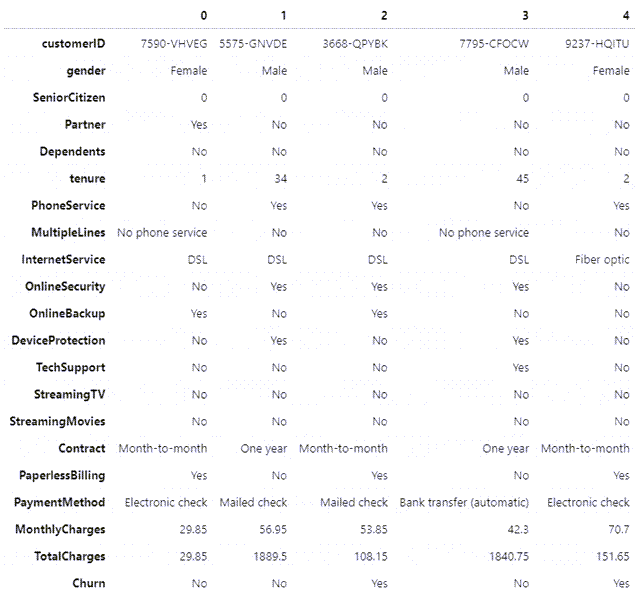2、 去除不需要的customerID列

data['customerID'].duplicated().any() #判断是否有重复
data = data.drop("customerID", axis=1) #去除列


3、 数据类型转化及去除空值行

data.TotalCharges = pd.to_numeric(data.TotalCharges, errors='coerce') #将字符串数值转化为数字
data.isnull().sum() #统计null值数量
data.dropna(inplace = True)


4、 显示类别型特征的类别情况

summary_categorical = []
for column in data.columns:
if data[column].dtype == object:
summary_categorical.append(column)
print(data[column].value_counts())
print(f"----------------------------------")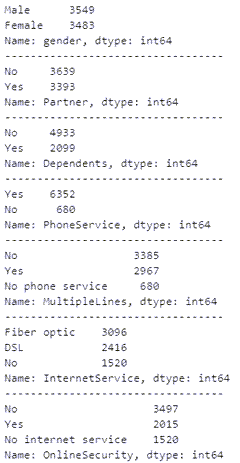data['gender'].replace(to_replace='Male', value=1, inplace=True)
data['gender'].replace(to_replace='Female',  value=0, inplace=True)

binary_features = ['Partner', 'Dependents', 'PhoneService','MultipleLines','OnlineSecurity', 'OnlineBackup', 'DeviceProtection', 'TechSupport',
'StreamingTV','StreamingMovies', 'PaperlessBilling','Churn']
for feat in binary_features:
data[feat].replace(to_replace='Yes', value=1, inplace=True)
data[feat].replace(to_replace=r'No',  value=0, regex=True, inplace=True)


data_dummies = pd.get_dummies(data)
data_dummies.info()


5、 查看特征间的相关性

import matplotlib.pyplot as plt
import seaborn as sns

corr = data_dummies.corr()
sns.heatmap(corr, xticklabels=corr.columns.values, yticklabels=corr.columns.values, annot = True, annot_kws={'size':12})
heat_map=plt.gcf()
heat_map.set_size_inches(20,15)
plt.xticks(fontsize=10)
plt.yticks(fontsize=10)
plt.show()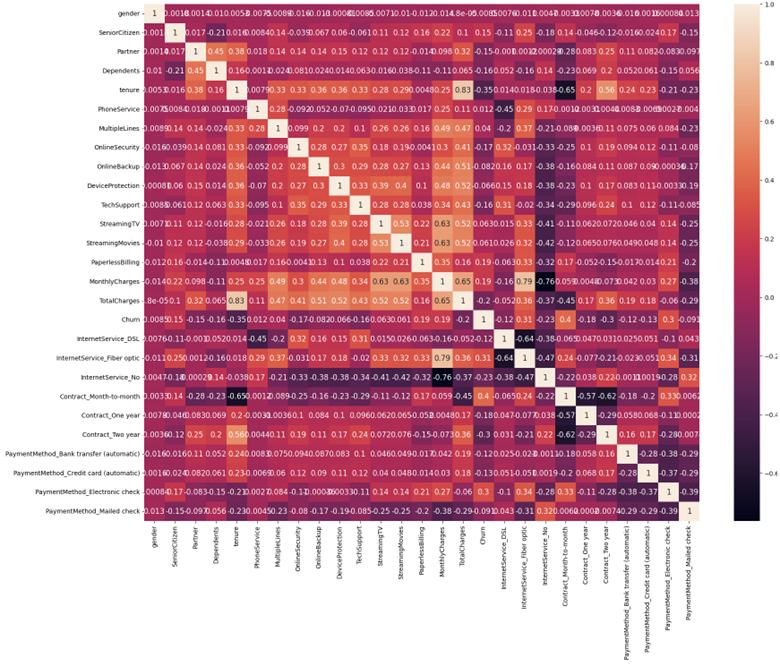# Checking again Correlation of "Churn" with other variables on a different plot
sns.set(style='darkgrid', context='talk', palette='Dark2')
plt.figure(figsize=(15,8))
data_dummies.corr()['Churn'].sort_values(ascending = False).plot(kind='bar')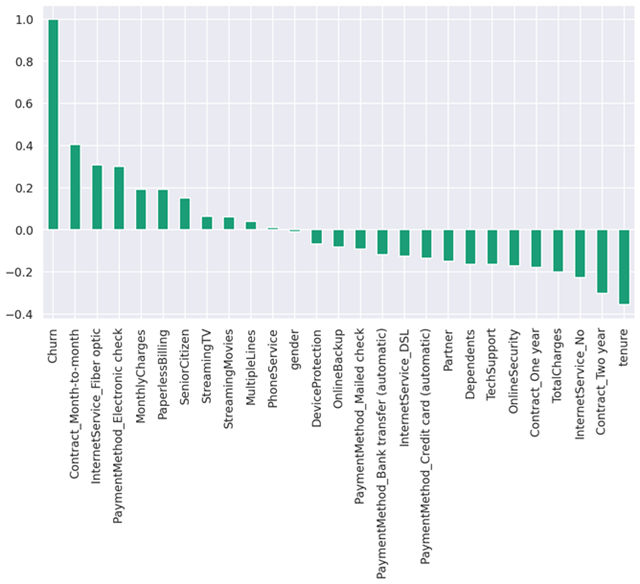columns_to_visualise = ['gender', 'SeniorCitizen', 'Partner', 'Dependents',
'PhoneService', 'MultipleLines', 'InternetService', 'OnlineSecurity',
'OnlineBackup', 'DeviceProtection', 'TechSupport', 'StreamingTV',
'StreamingMovies', 'Contract', 'PaperlessBilling', 'PaymentMethod']

for column in columns_to_visualise:
plot_data = data.groupby([column, 'Churn']).size().reset_index().pivot(columns='Churn', index=column, values=0)
plot_data.plot.bar(stacked=True, rot = 45)
plt.show()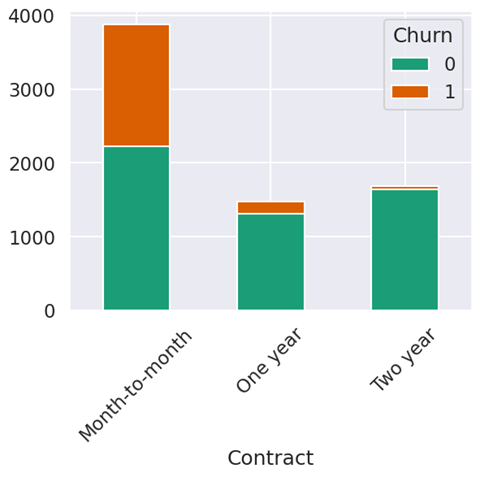# Visualise the numerical features
fig, (ax1, ax2, ax3) = plt.subplots(1, 3, figsize = (20,6))
fig.suptitle("KDE of continuous feature variables")
# tenure
sns.kdeplot(data['tenure'].loc[data['Churn'] == 0], label='not churn', fill=True, ax = ax1)
sns.kdeplot(data['tenure'].loc[data['Churn'] == 1], label='churn', fill=True, ax = ax1)
ax1.set_xlabel("Tenure in months")
# monthly charges
sns.kdeplot(data['MonthlyCharges'].loc[data['Churn'] == 0], label='not churn', fill=True, ax = ax2)
sns.kdeplot(data['MonthlyCharges'].loc[data['Churn'] == 1], label='churn', fill=True, ax = ax2)
ax2.set_xlabel("Monthly Charges ($)") # total charges sns.kdeplot(data['TotalCharges'].loc[data['Churn'] == 0], label='not churn', fill=True, ax = ax3) sns.kdeplot(data['TotalCharges'].loc[data['Churn'] == 1], label='churn', fill=True, ax = ax3) ax3.set_xlabel("Total Charges ($)")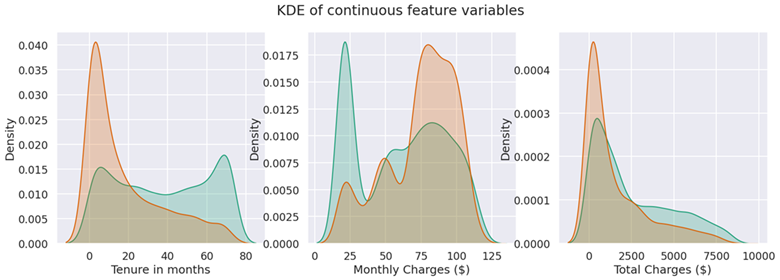# Check for any outliers in any of the continuous variables
continuous_labels = ['tenure', 'MonthlyCharges', 'TotalCharges']
i = 1
plt.figure(figsize=(15,15))
for var in continuous_labels: #plotting boxplot for each variable
plt.subplot(3,4,i)
plt.boxplot(data[var],whis=5)
plt.title(var)
i+=1
plt.tight_layout()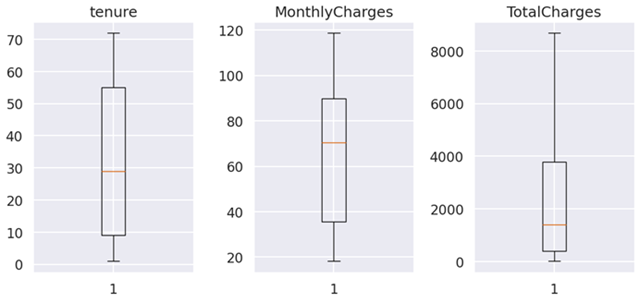continuous_labels = ['tenure', 'MonthlyCharges', 'TotalCharges']
for var in continuous_labels:
sns.boxplot(x = data['Churn'], y = data[var])
plt.show()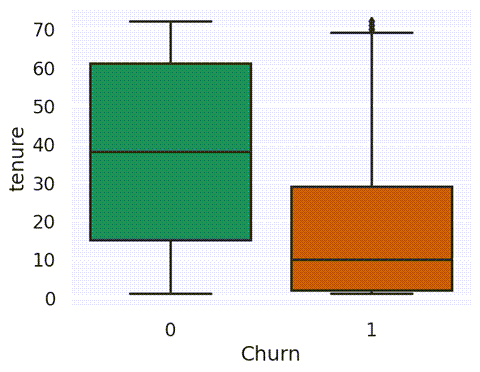# Visualise scatter plots of tenure against monthly and total charges based on churn
fig, (ax1, ax2) = plt.subplots(1, 2, figsize = (20,6))
fig.suptitle("Scatter plots of continuous feature variables")

# Monthly Charges
ax1.scatter(data['tenure'], data['MonthlyCharges'], c=data['Churn'])
ax1.set_xlabel('Customer Tenure (Months)')
ax1.set_ylabel('Monthly Charges')

# Total Charges
ax2.scatter(data['tenure'], data['TotalCharges'], c=data['Churn'])
ax2.set_xlabel('Customer Tenure (Months)')
ax2.set_ylabel('Total Charges');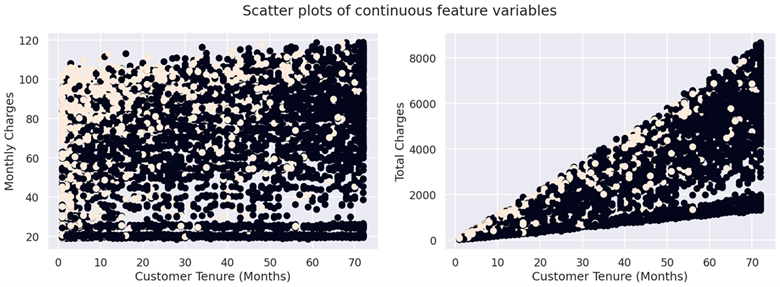import matplotlib.pyplot as plt

time = data['tenure'].sample(25, replace=False)
status = data['Churn'].sample(25, replace=False)

plt.figure(figsize=(16, 6));
plt.xlabel('Days subscribed');
plt.ylabel('Customer ID');
plt.title('Customer subscription lifelines')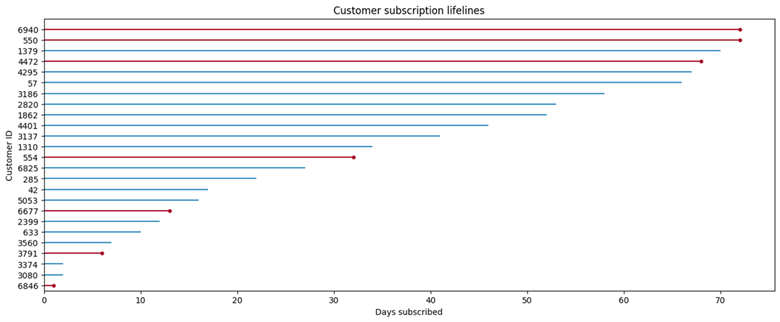### 使用逻辑回归进行流失预测

1、准备数据并进行逻辑回归

# Using the data frame where we had created dummy variables
y = data_dummies['Churn'].values
X = data_dummies.drop(columns = ['Churn'])

# Scaling all the variables to a range of 0 to 1
from sklearn.preprocessing import MinMaxScaler
features = X.columns.values
scaler = MinMaxScaler(feature_range = (0,1))
scaler.fit(X)
X = pd.DataFrame(scaler.transform(X))
X.columns = features

from sklearn.model_selection import train_test_split

X_train, X_test, y_train, y_test = train_test_split(X, y, test_size=0.2, random_state=101, stratify=y)
print('length of X_train and x_test: ', len(X_train), len(X_test))
print('length of y_train and y_test: ', len(y_train), len(y_test))

from sklearn.linear_model import LogisticRegression
from sklearn.metrics import confusion_matrix, accuracy_score
from sklearn import metrics

model = LogisticRegression(solver='lbfgs', max_iter=1000)
result = model.fit(X_train, y_train)
y_pred = model.predict(X_test)

print ("Prdiction:",metrics.accuracy_score(y_test, y_pred))
print("Precision:",metrics.precision_score(y_test, y_pred))
print("Recall:",metrics.recall_score(y_test, y_pred))

print('Intercept: ' + str(result.intercept_)) # reporting the intercept
print('Regression: ' + str(result.coef_)) # reporting the co-efficients


2、查看模型的质量

import itertools
import numpy as np

#Evaluation of Model - Confusion Matrix Plot
def plot_confusion_matrix(cm, classes, title ='Confusion matrix', normalize = False, cmap = plt.cm.Blues):
"""
This function prints and plots the confusion matrix.
Normalization can be applied by setting normalize=True.
"""
if normalize:
cm = cm.astype('float') / cm.sum(axis=1)[:, np.newaxis]
print("Normalized confusion matrix")
else:
print('Confusion matrix')

print(cm)

plt.imshow(cm, interpolation='nearest', cmap=cmap)
plt.title(title)
plt.colorbar()
tick_marks = np.arange(len(classes))
plt.xticks(tick_marks, classes, rotation=45)
plt.yticks(tick_marks, classes)

fmt = '.2f' if normalize else 'd'
thresh = cm.max() / 2.
for i, j in itertools.product(range(cm.shape), range(cm.shape)):
plt.text(j, i, format(cm[i, j], fmt),
horizontalalignment="center",
color="white" if cm[i, j] > thresh else "black")

plt.ylabel('True label')
plt.xlabel('Predicted label')
plt.tight_layout()

# Compute confusion matrix
cnf_matrix = confusion_matrix(y_test, prediction_test)
np.set_printoptions(precision=2)

# Plot non-normalized confusion matrix
plt.figure()
plot_confusion_matrix(cnf_matrix, classes=['No Churn','Churn'], title='Confusion matrix, with normalized data')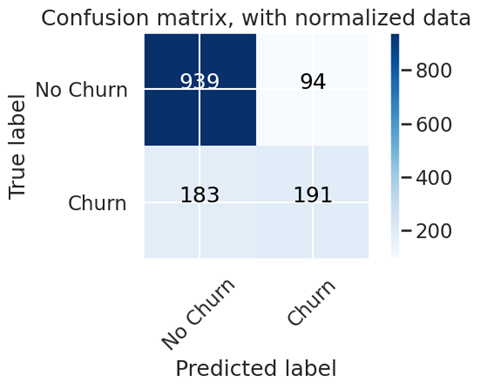# To get the weights of all the variables
weights = pd.Series(model.coef_,index=X.columns.values)
weights.sort_values(ascending = False)


#!pip install scikit-plot
import scikitplot as skplt #to make things easy
y_pred_proba = model.predict_proba(X_test)
skplt.metrics.plot_roc_curve(y_test, y_pred_proba)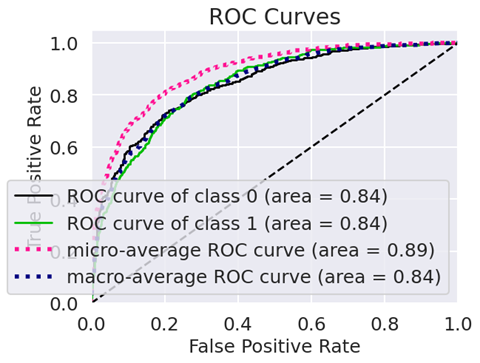3、将数据调整到平衡后再进行一轮模型测试

data_dummies['Churn'].value_counts()

from sklearn.utils import resample

data_majority = data_dummies[data_dummies['Churn']==0]
data_minority = data_dummies[data_dummies['Churn']==1]

data_minority_upsampled = resample(data_minority, replace=True,
n_samples=5163, #same number of samples as majority class
random_state=1) #set the seed for random resampling

# Combine resampled results
data_upsampled = pd.concat([data_majority, data_minority_upsampled])
data_upsampled['Churn'].value_counts()

from sklearn.metrics import classification_report

train, test = train_test_split(data_upsampled, test_size = 0.20)

train_y_upsampled = train['Churn'].values
test_y_upsampled = test['Churn'].values

train_x_upsampled = train.drop(columns = ['Churn'])
test_x_upsampled = test.drop(columns = ['Churn'])

logisticRegr_balanced = LogisticRegression(solver='lbfgs', max_iter=1000)
logisticRegr_balanced.fit(X=train_x_upsampled, y=train_y_upsampled)

test_y_pred_balanced = logisticRegr_balanced.predict(test_x_upsampled)

# ! pip install yellowbrick

from sklearn.naive_bayes import GaussianNB
from yellowbrick.classifier import ClassificationReport

classes=['Churn','No Churn']

# Instantiate the classification model and visualizer
bayes = GaussianNB()
visualizer = ClassificationReport(bayes, classes=classes, support=True)

visualizer.fit(train_x_upsampled, train_y_upsampled)  # Fit the visualizer and the model
visualizer.score(test_x_upsampled, test_y_upsampled)  # Evaluate the model on the test data
g = visualizer.poof()             # Draw/show/poof the data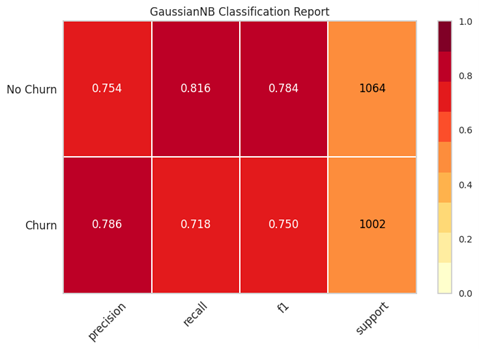from sklearn.metrics import roc_auc_score

# Get class probabilities for both models
test_y_prob = model.predict_proba(X_test)
test_y_prob_balanced = model.predict_proba(test_x_upsampled)

# We only need the probabilities for the positive class
test_y_prob = [p for p in test_y_prob]
test_y_prob_balanced = [p for p in test_y_prob_balanced]

print('Unbalanced model AUROC: ' + str(roc_auc_score(y_test, test_y_prob)))
print('Balanced model AUROC: ' + str(roc_auc_score(test_y_upsampled, test_y_prob_balanced)))

from sklearn.model_selection import cross_val_score

# evaluate the model using 10-fold cross-validation
scores = cross_val_score(result, X_train, y_train, scoring='accuracy', cv=10)
print (' 10 fold cross-validation scores: ' ,scores)
print('Mean of scores: ', scores.mean())


OLS 通过绘制最小化平方误差项总和的回归线来工作。然而，对于未观察到的数据，无法知道误差项，因此不可能最小化这些值。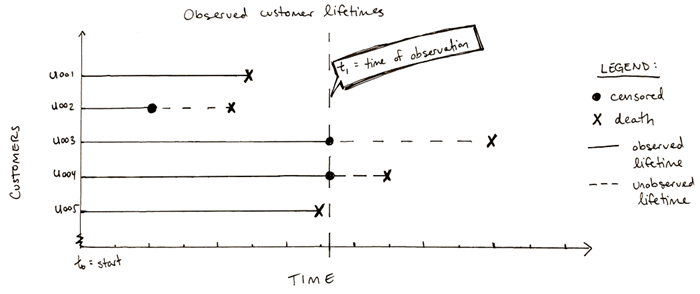• “出生”事件：对于我们的应用程序，这将是客户与公司签订合同
• “死亡”事件：对我们而言，“死亡”是客户结束与公司的关系

### Kaplan-Meier(KM)生存分析

Kaplan 是完全参数化的，也是计算生存或“无流失”的最简单方法。Kaplan-Meier 生存曲线定义为在给定时间长度内“没有流失”的概率，同时考虑许多小区间的时间。对于每个时间间隔，“无流失”概率计算为保留的用户数除以有离开风险的用户数。

Kaplan-Meier的优点：

• 需要最少的功能集。Kaplan-Meier 只需要事件发生的时间（死亡或审查）以及出生和事件之间的生命周期。
• 许多时间序列分析难以实施。Kaplan-Meier 只需要所有事件都在同一时间段内发生
• 自动处理类别不平衡（死亡与审查事件的任何比例都可以）
• 因为它是一种非参数方法，所以很少对数据的基本分布做出假设

Kaplan-Meier的缺点：

• 无法估计感兴趣的生存预测关系的差异幅度（无风险比或相对风险）
• 在事件发生时间研究中，不能同时考虑每个受试者的多个因素，也不能控制混杂因素
• 假设审查和生存之间是独立的，这意味着在时间t，那些被审查的人应该与那些没有被审查的人有相同的预后。
• 因为它是一个非参数模型，所以在底层数据分布已知的问题上，它不如竞争技术那么有效或准确

import lifelines
from lifelines import KaplanMeierFitter

# fitting kmf to churn data
t = data['tenure']
churn = data['Churn']
kmf = lifelines.KaplanMeierFitter()
kmf.fit(t, churn, label='Estimate for Average Customer')

# plotting kmf curve
fig, ax = plt.subplots(figsize=(10,7))
kmf.plot(ax=ax)
ax.set_title('Kaplan-Meier Survival Curve — All Customers')
ax.set_xlabel('Customer Tenure -Months')
ax.set_ylabel('Customer Survival Chance (%)')
plt.show()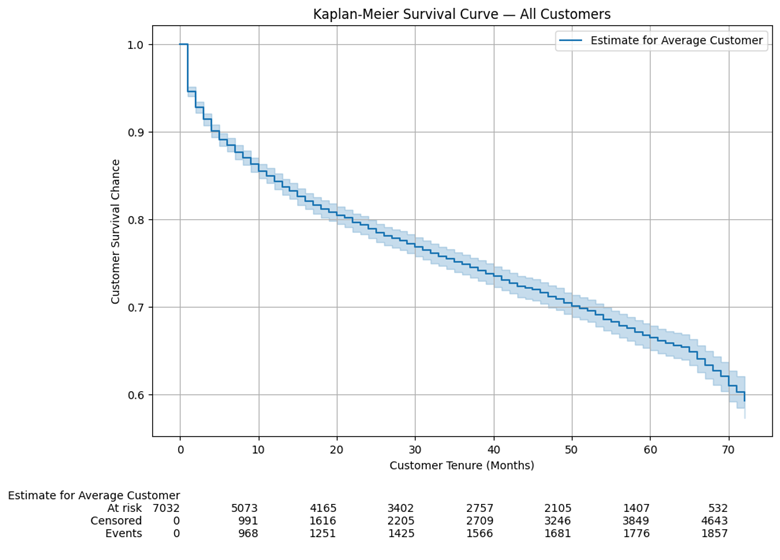• 处于风险中：观察到的任期超过该时间点的客户数量。例如，532 位客户的任期超过 70 个月
• 已审查：未流失的任期等于或小于该时间点的客户数量。例如，3,860 名客户的任期为 60 个月或更短，但他们当时并没有流失
• 事件：使用期限等于或小于该时间点的客户数量，该时间点已经流失。例如，1,681 名客户的任期为 50 个月或更短，并且在那时已经流失

# save indices for each contract type
idx_m2m = data['Contract'] == 'Month-to-month'
idx_1y = data['Contract'] == 'One year'
idx_2y = data['Contract'] == 'Two year'

# plot the 3 KM plots for each category
fig, ax = plt.subplots(nrows = 1, ncols = 1, figsize = (10,10))
kmf_m2m = lifelines.KaplanMeierFitter()
ax = kmf_m2m.fit(durations = data.loc[idx_m2m, 'tenure'], event_observed = data.loc[idx_m2m, 'Churn'], label = 'Month-to-month').plot(ax = ax)
kmf_1y = lifelines.KaplanMeierFitter()
ax = kmf_1y.fit(durations = data.loc[idx_1y, 'tenure'], event_observed = data.loc[idx_1y, 'Churn'], label = 'One year').plot(ax = ax)
kmf_2y = lifelines.KaplanMeierFitter()
ax = kmf_2y.fit(durations = data.loc[idx_2y, 'tenure'], event_observed = data.loc[idx_2y, 'Churn'], label = 'Two year').plot(ax = ax)

# display title and labels
ax.set_title('KM Survival Curve by Contract Duration')
ax.set_xlabel('Customer Tenure (Months)')
ax.set_ylabel('Customer Survival Chance')
plt.grid()
lifelines.plotting.add_at_risk_counts(kmf_m2m, kmf_1y, kmf_2y, ax = ax)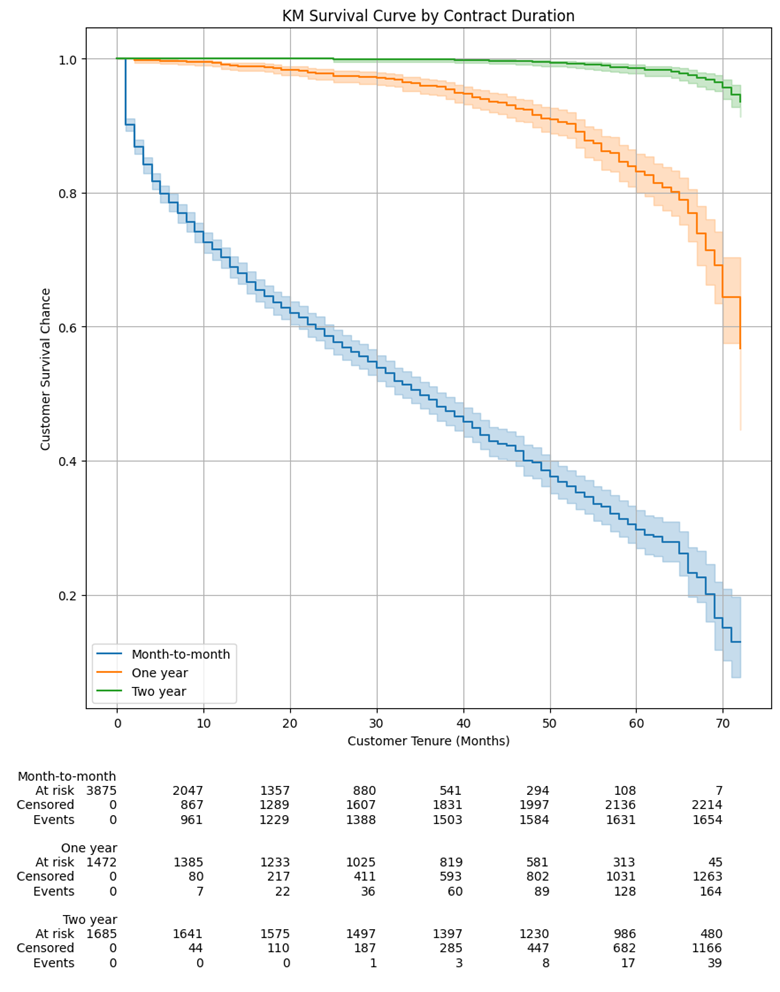• 签订 2 年合同的客户几乎有 100% 的生存概率
• 签订 1 年合同的客户有超过 95% 的生存概率
• 每月合同的客户只有大约 45% 的生存概率

### Cox模型

1、应用模型并显示模型效果

Cox模型就像 scikit-learn 中的模型一样通过模型的print_summary方法访问以下模型摘要：

# 去掉共线特征
data_dummies.drop(columns = ['InternetService_Fiber optic', 'Contract_Month-to-month', 'PaymentMethod_Electronic check'], inplace = True)
data.info()

# Instantiate and fit CPH model
cph = lifelines.CoxPHFitter()
cph.fit(data_dummies, duration_col = 'tenure', event_col = 'Churn')

# Print model summary
cph.print_summary(model = 'base model', decimals = 3, columns = ['coef', 'exp(coef)', 'p'])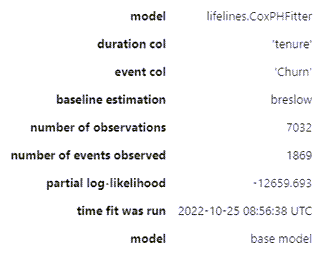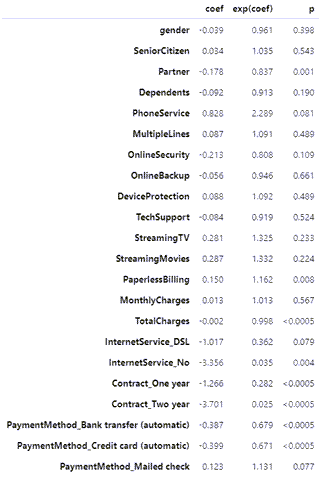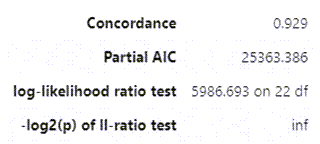• 模型系数（coef列）告诉我们每个协变量如何影响风险。协变量为正coef表示具有该特征的客户更有可能流失，反之亦然
• exp(coef)是风险比，解释为变量每增加一个单位的风险比例，00 是中性的。例如，1.325 的风险比StreamingMovies意味着订阅流媒体电影服务的客户取消其服务的可能性高出 32.5%。从我们的角度来看，exp(coef)低于 1.0 是好的，这意味着客户在存在该协变量的情况下不太可能取消
• 模型一致性929 的解释与逻辑回归的 AUROC 类似：
• 接近5 是随机预测的预期结果
• 越接近0，1.0 显示出完美的预测一致性越好。

0.929 的一致性基本上意味着我们的模型在未经审查的数据上正确预测了 100 对中的92.9对。它基本上评估了模型的区分能力，即它在区分活着的和流失的对象方面有多好。比较两个不同的模型很有用。然而，concordance 并没有说明我们的模型校准得有多好——我们稍后会评估这一点。

2、确认比例危害 (PH) 假设

# Check model assumptions, with a threshold of 0.001 (i.e. only highlight extreme significances - rationale explained after the results)
cph.check_assumptions(data_dummies, p_value_threshold=0.001, show_plots=True)

The p_value_threshold is set at 0.001. Even under the null hypothesis of no violations, some
covariates will be below the threshold by chance. This is compounded when there are many covariates.
Similarly, when there are lots of observations, even minor deviances from the proportional hazard
assumption will be flagged.

With that in mind, it's best to use a combination of statistical tests and visual tests to determine
the most serious violations. Produce visual plots using check_assumptions(..., show_plots=True)
and looking for non-constant lines. See link [A] below for a full example.

 null_distribution chi squared degrees_of_freedom 1 model
1. Variable 'TotalCharges' failed the non-proportional test: p-value is <5e-05.

Advice 1: the functional form of the variable 'TotalCharges' might be incorrect. That is, there
may be non-linear terms missing. The proportional hazard test used is very sensitive to incorrect
functional forms. See documentation in link [D] below on how to specify a functional form.

Advice 2: try binning the variable 'TotalCharges' using pd.cut, and then specify it in
strata=['TotalCharges', ...] in the call in .fit. See documentation in link [B] below.

below.

Bootstrapping lowess lines. May take a moment...

2. Variable 'Contract_One year' failed the non-proportional test: p-value is <5e-05.

Advice: with so few unique values (only 2), you can include strata=['Contract_One year', ...]
in the call in .fit. See documentation in link [E] below.

Bootstrapping lowess lines. May take a moment...

3. Variable 'Contract_Two year' failed the non-proportional test: p-value is <5e-05.

Advice: with so few unique values (only 2), you can include strata=['Contract_Two year', ...]
in the call in .fit. See documentation in link [E] below.

Bootstrapping lowess lines. May take a moment...

---

:
[[<AxesSubplot: xlabel='rank-transformed time\n(p=0.0000)'>,
<AxesSubplot: xlabel='km-transformed time\n(p=0.0000)'>],
[<AxesSubplot: xlabel='rank-transformed time\n(p=0.0000)'>,
<AxesSubplot: xlabel='km-transformed time\n(p=0.0000)'>],
[<AxesSubplot: xlabel='rank-transformed time\n(p=0.0000)'>,
<AxesSubplot: xlabel='km-transformed time\n(p=0.0000)'>]]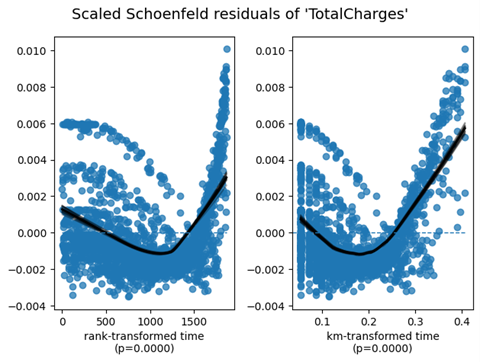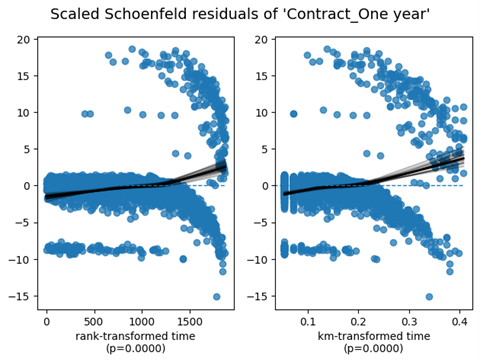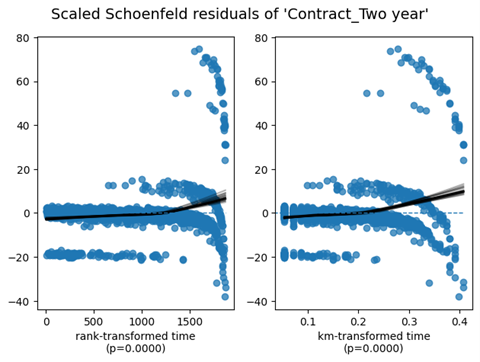3、CPH 模型验证

lifelines库有一个内置函数来执行我们拟合模型的 k 折交叉验证。使用一致性指数作为评分参数运行它会导致十倍的平均一致性指数为 0.928。

Concordance Index 没有说明模型的校准——即预测概率与实际真实概率的差距有多大？我们可以绘制一条校准曲线来检查我们的模型在任何给定时间正确预测概率的倾向。sklearn现在让我们使用’calibration_curve函数在 t = 12 处绘制校准曲线：

from sklearn.calibration import calibration_curve

plt.figure(figsize=(10, 10))

ax1 = plt.subplot2grid((3, 1), (0, 0), rowspan=2)

# Plot the perfectly calibrated line with 0 intercept and 1 slope
ax1.plot([0, 1], [0, 1], ls = '--', label = 'Perfectly calibrated')

# Calculate the churn probabilities at the end of 12th month. predict_survival_function gives us the survival probability, which we have deducted from 1 to get the churn probability
probs = 1 - np.array(cph.predict_survival_function(data_dummies, times = 12).T)

actual = data['Churn']
# For each decile, the calibration curve will plot the mean predicted churn probability on the x-axis and its corresponding proportion of observations that actually churned on y-axis, in each bin
fraction_of_positives, mean_predicted_value = calibration_curve(actual, probs, n_bins = 10, strategy = 'quantile')
ax1.plot(mean_predicted_value, fraction_of_positives, marker = 's', ls = '-', label='CoxPH')

ax1.set_ylabel("Actual fraction of positives")
ax1.set_xlabel("Predicted churn probability")
ax1.set_ylim([-0.05, 1.05])
ax1.legend(loc="lower right")
ax1.set_title('Calibration plots (reliability curve) for the 12th month')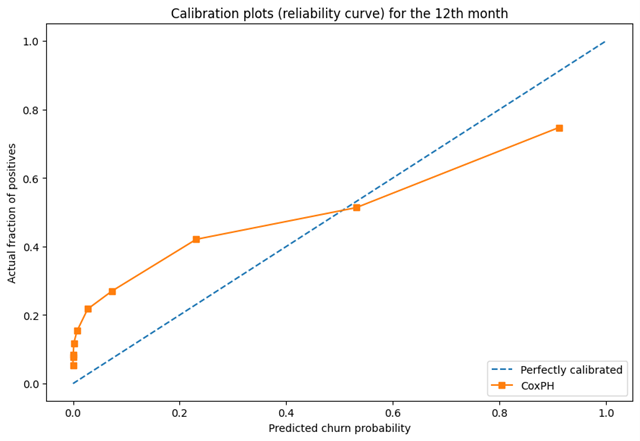from sklearn.metrics import brier_score_loss

# calculate Brier Score
brier_score = brier_score_loss(data_dummies['Churn'], 1 - np.array(cph.predict_survival_function(data_dummies, times = 12).T), pos_label = 1)
print('The Brier Score of our CPH Model is {:.2f} at the end of 12 months'.format(brier_score))

brier_score_dict = {}
# Loop over all the months
for i in range(1,73):
score = brier_score_loss(data_dummies['Churn'], 1 - np.array(cph.predict_survival_function(data_dummies, times = i).T), pos_label=1)
brier_score_dict[i] = [score]
# Convert the dict to a DF
brier_score_df = pd.DataFrame(brier_score_dict).T
# Plot the Brier Score over time
fig, ax = plt.subplots()
ax.plot(brier_score_df)
ax.set(xlabel='Month', ylabel='Brier Score', title='Cox PH Model Calibration Over Time')
ax.grid()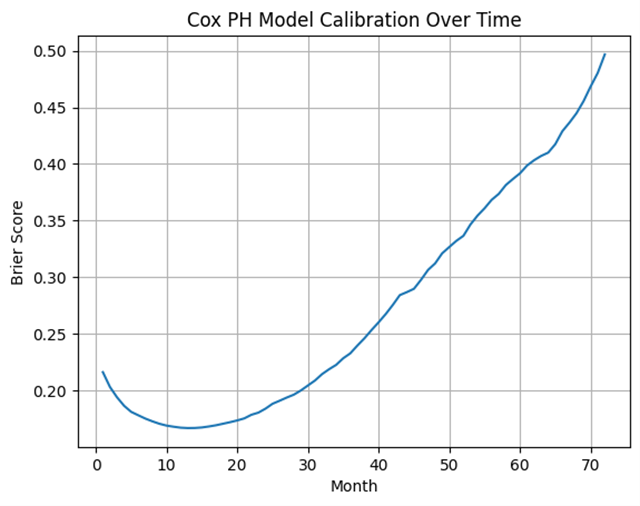4、CPH 模型可视化

# Let's plot the coefficient outputs and their respective confidence intervals
fig_coef, ax_coef = plt.subplots(figsize = (12,7))
ax_coef.set_title('Survival Regression: Coefficients and Confidence Intervals')
cph.plot(ax = ax_coef)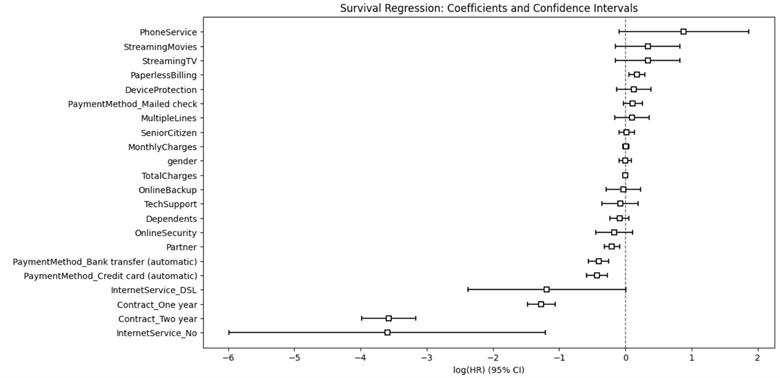• PhoneService
• StreamingMovies
• StreamingTV
• PaperlessBilling
• DeviceProtection
• PaymentMothod_Mailed check

• intertnetService_No
• Contact_Two Year
• Contact_One Year
• intertnetService_DSL
• PaymentMothod_Credit card(automatic)
• PaymentMothod_Bank transfer(automatic)

# Define figure and axes
fig, (ax1, ax2) = plt.subplots(nrows = 1, ncols = 2, figsize = (20,9))
# Total Charges
cph.plot_partial_effects_on_outcome('TotalCharges', values = [0, 3000, 6000], ax = ax1)
ax1.set_title('CPH Survival Curve by Total Charges')
ax1.set_xlabel('Tenure (Months)')
ax1.set_ylabel('Survival Chance (%)')

# Contract
cph.plot_partial_effects_on_outcome(['Contract_One year', 'Contract_Two year'], values = [[1, 0], [0, 1]], ax = ax2) # we have two arrays in values, 1 for each of the covariate. eq to np.eye(2)
ax2.set_title('CPH Survival Curve by Contract Duration')
ax2.set_xlabel('Tenure (Months)')
ax2.set_ylabel('Survival Chance (%)')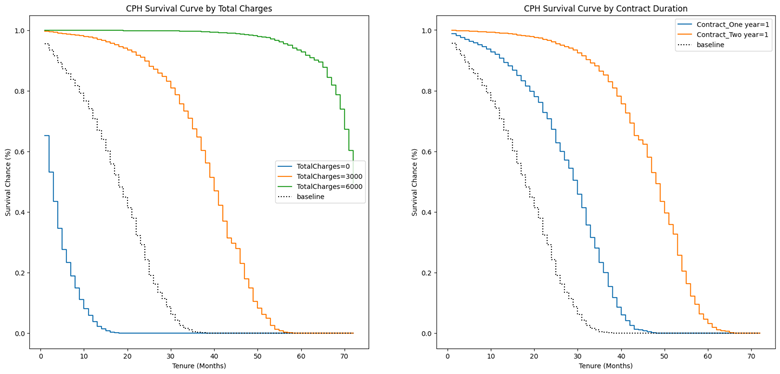4、客户流失预测和预防

kmf = lifelines.KaplanMeierFitter()
# We will use the data_kmf that we kept aside for this moment before feature engineering

# function for creating KM curves segmented by categorical variables
def plot_categorical_KM_Curve(feature, t='tenure', event='Churn', df=data, ax=None):
for cat in df[feature].unique():
idx = df[feature] == cat
kmf.fit(df[idx][t], event_observed=df[idx][event], label=cat)
kmf.plot(ax=ax, label=cat)

# call the above function and plot 4 KM Curves
fig, ((ax1, ax2), (ax3, ax4)) = plt.subplots(nrows = 2, ncols = 2, figsize=(20,10))
# PaymentMethod
plot_categorical_KM_Curve(feature='PaymentMethod', ax=ax1)
ax1.set_title('Kaplan-Meier Survival Curve by Payment Method')
ax1.set_xlabel('Customer Tenure (Months)')
ax1.set_ylabel('Customer Survival Chance (%)')

# Contract
plot_categorical_KM_Curve(feature='Contract', ax=ax2)
ax2.set_title('Kaplan-Meier Survival Curve by Contract Duration')
ax2.set_xlabel('Customer Tenure (Months)')
ax2.set_ylabel('Customer Survival Chance (%)')

# InternetService
plot_categorical_KM_Curve(feature='InternetService', ax=ax3)
ax3.set_title('Kaplan-Meier Survival Curve by Internet Service')
ax3.set_xlabel('Customer Tenure (Months)')
ax3.set_ylabel('Customer Survival Chance (%)')

# PhoneService
plot_categorical_KM_Curve(feature='PhoneService', ax=ax4)
ax4.set_title('Kaplan-Meier Survival Curve by Phone Service')
ax4.set_xlabel('Customer Tenure (Months)')
ax4.set_ylabel('Customer Survival Chance (%)')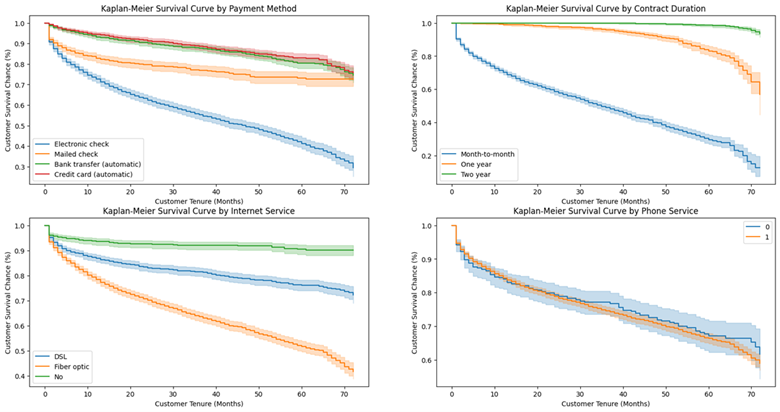• 鼓励客户通过银行转帐或信用卡设置自动付款
• 让客户签订 1 年或 2 年的合同
• 关于互联网服务，首先我们应该分析一下DSL或光纤互联网订阅用户流失率较高的根本原因——可能是服务质量差、价格高、客户服务不足等。我们也应该这样做互联网服务盈利能力分析。最佳行动方案取决于该分析。
• 有或没有电话服务的客户的生存曲线似乎没有太大的统计差异

5、分析审查客户

predict_survival_function的输出将是一个矩阵，其中包含每个剩余客户在特定未来时间点的生存概率，直至我们数据中的最大历史持续时间。因此，如果我们的数据中的最长持续时间是 70 个月，predict_survival_function那么将从今天开始预测接下来的 70 个月。该方法还允许我们通过参数计算未来特定月份的生存概率（有助于回答诸如我们希望在接下来的 3 个月和 6 个月末保留多少客户等问题）

censored_data = data_dummies[data_dummies['Churn'] == 0]
censored_data_last_obs = censored_data['tenure']
conditioned_sf = cph.predict_survival_function(censored_data, conditional_after = censored_data_last_obs)
conditioned_sf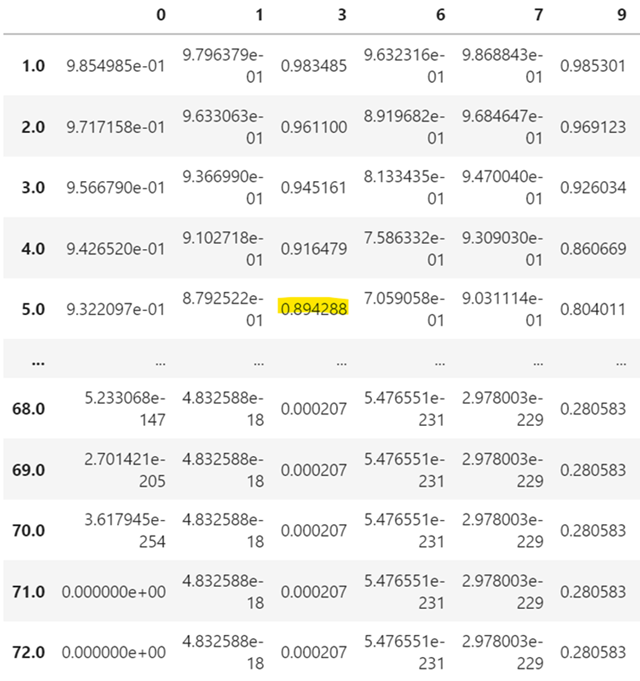6、计算预期损失

# Predict the month where the survival probability falls below the median
predictions_50 = lifelines.utils.median_survival_times(conditioned_sf)
predictions_50
# Use the following if we wanted to assign any other threshold, i.e. month when the survival function reaches the qth percentile
# predictions_25 = lifelines.utils.qth_survival_times(0.25, conditioned_sf) # 25% survival chance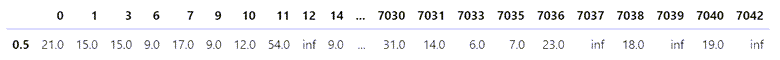inf基本上意味着这个客户几乎可以肯定还活着。

• 将转置后的输出median_survival_times与 CustomerID 和各自的 MonthlyCharges（我们一开始就放在一边）连接起来。这将使我们能够将我们的预测与每个特定客户及其每月费用联系起来。
• 通过将每月费用乘以他们的预期流失月份来计算每个客户在今天流失时的预期损失。
• 对于inf预期流失月份的客户，替换inf为启发式，可能是 24，对应于 2 年的合同。如果他们今天离开我们，假设他们将在我们身边至少呆 24 个月，这将使我们能够估计这些客户的相关预期损失。

# We can use this single row and by joining it to our data DF, we can investigate the predicted remaining value a customer has for the business
# Note that we will also append our censored customerIDs that we kept aside at the beginning of the model so as to properly identify our customers
customer_predictions = pd.concat([customerID[['customerID', 'MonthlyCharges']], predictions_50.T], axis = 1)

# Rename the column returned by median_survival_times function
customer_predictions.rename(columns = {0.5: 'Exp_Churn_Month'}, inplace = True)

# Add another column for the expected loss if these customers were to leave us today
customer_predictions['Exp_Loss'] = customer_predictions['MonthlyCharges'] * customer_predictions['Exp_Churn_Month']
customer_predictions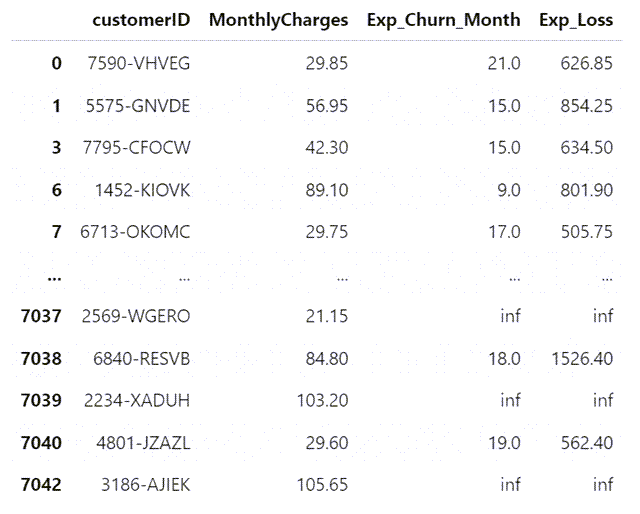# Assign 24 to inf values in Exp_Churn_Month
customer_predictions['Exp_Churn_Month'].replace([np.inf, -np.inf], 24, inplace = True)

# Recalculate the customer_predictions table and sort it
customer_predictions['Exp_Loss'] = customer_predictions['MonthlyCharges'] * customer_predictions['Exp_Churn_Month']
customer_predictions.sort_values(by = ['Exp_Loss'], ascending = False)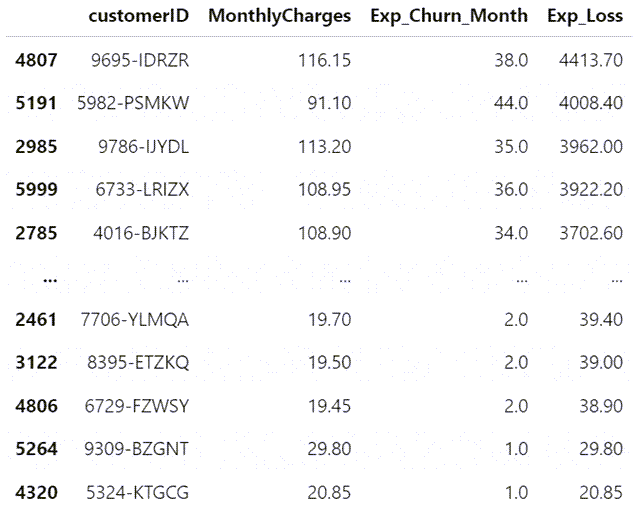7、计算预计收入提升

• 1年的合同
• 2年的合同
• DSL
• 通过银行转账自动付款
• 通过信用卡自动付款

# Store the column names to be analysed in a list
upgrades = ['Contract_One year', 'Contract_Two year', 'InternetService_DSL', 'PaymentMethod_Bank transfer (automatic)', 'PaymentMethod_Credit card (automatic)']
# Define an empty dictionary to hold the results
results_dict = {}

# For each of the potential upgrades, loop through each individual customer to determine the increase in expected median churn month
for customer in customer_predictions.index:
actual = censored_data.loc[[customer]] # save the actual cutomer data as a series
change = censored_data.loc[[customer]] # same as actual but this series will be used to evaluate hypothetical scenarios
results_dict[customer] = [cph.predict_median(actual, conditional_after=censored_data_last_obs[customer])] # calculate the base median churn month
change[upgrade] = 1 if list(change[upgrade]) == 0 else 1 # hypothetical scenario where customer signs up for this particular upgrade
results_dict[customer].append(cph.predict_median(change, conditional_after = censored_data_last_obs[customer])) # calculate the revised median churn month under the above hypothetical scenario
change = censored_data.loc[[customer]] # bring the change series back to the original state (i.e. undo the effect of the hypothetical scenario)

# Convert dictionary to a DF and transpose the resultant DF back to the required format (each customer in a separate row)
results_df = pd.DataFrame(results_dict).T

# add 'baseline' to the beginning of upgrades list. This new list will be used to rename the columns of results_df
column_names.insert(0, 'baseline')
results_df.columns = column_names

# Concat this new df with customer_predictions DF
upgrade_analysis = pd.concat([customer_predictions, results_df], axis = 1)
upgrade_analysis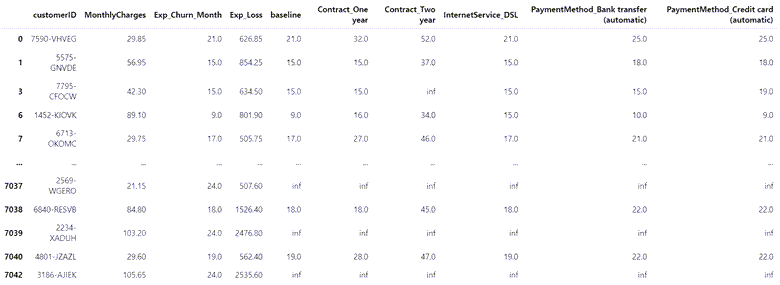# replace inf values in baseline column with NaN before dropping these rows
upgrade_analysis['baseline'].replace([np.inf, -np.inf], np.nan, inplace = True)
upgrade_analysis.dropna(subset = ['baseline'], axis=0, inplace = True)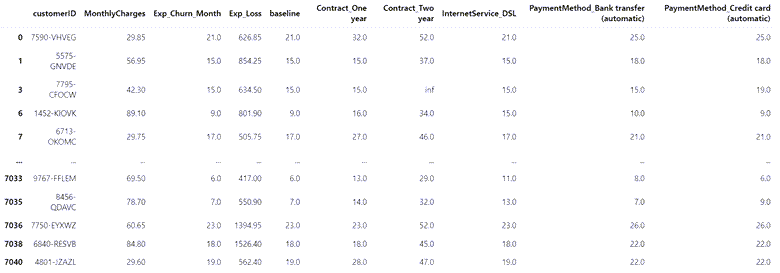# Calculate the difference in months between baseline and each feature's revised tenures and multiple this difference by MonthlyCharges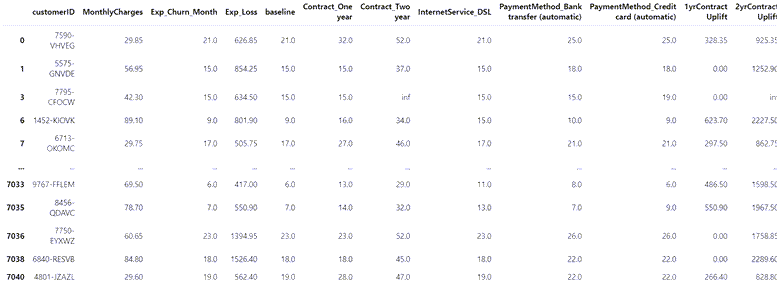• 如果他签署一份为期一年的合同，在其他条件不变的情况下，他预计会增加35 美元的额外收入
• 如果他签署一份为期 2 年的合同，在其他条件不变的情况下，他的预期寿命将增加35 美元
• 如果他转换为其中一种自动付款方式，在其他条件不变的情况下，他的预期寿命将增加4 美元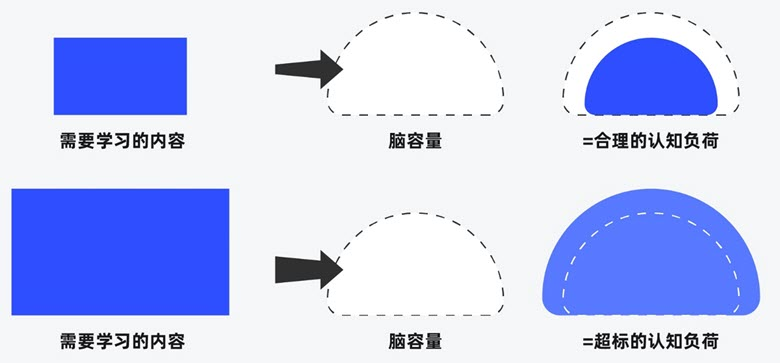##### 界面设计和游戏设计中的认知负荷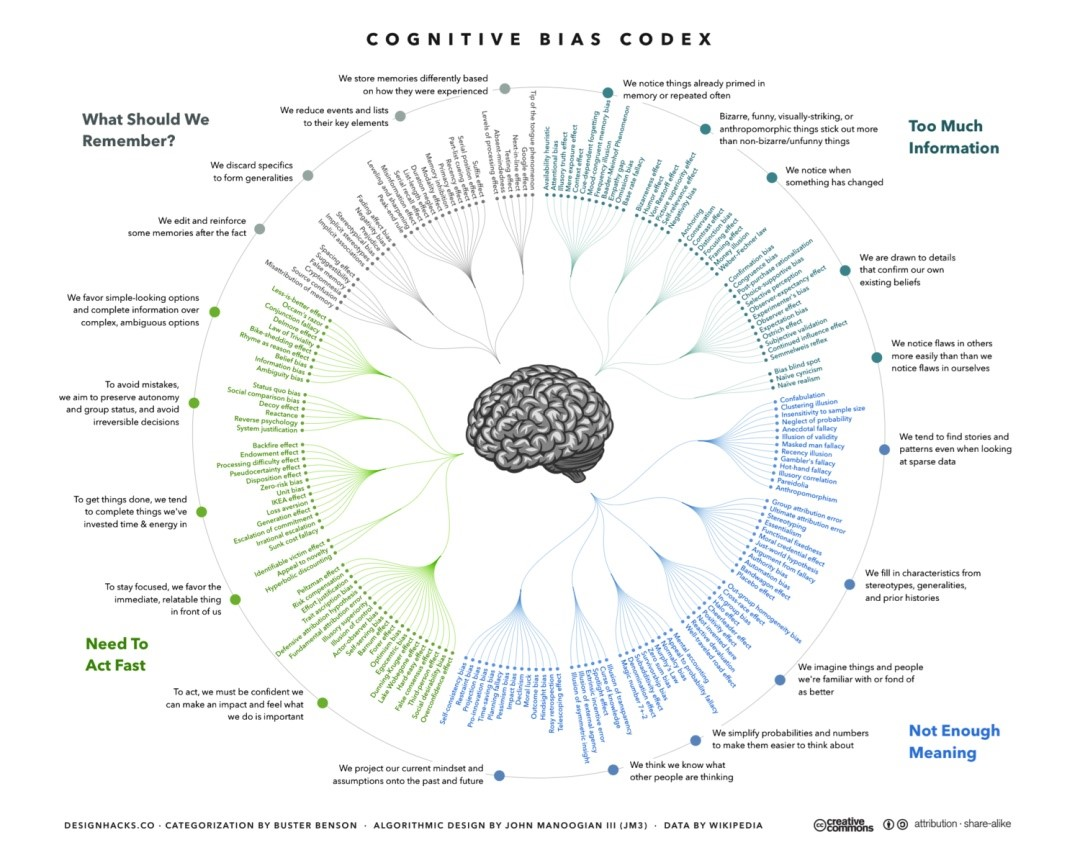##### 理解认知偏差